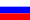# Vestnik KRAUNC. Fiz.-Mat. nauki. 2022. vol. 39. no. 2. P. 32-41. ISSN 2079-6641

Contents of this issue

Read Russian VersionMSC 35J15

Research Article

On one boundary value problem for the fourth-order equation in partial derivatives

O. Sh. Kilichov¹, A. N. Ubaydullaev²

¹V. I. Romanovskiy Institute of Mathematics, Uzbekistan Academy of Sciences, 4b University str., Tashkent, 100174, Uzbekistan
²Bukhara State University, M. Ikbol str. 11, Bukhara, 705018, Uzbekistan

E-mail: oybek2402@mail.ru

The initial-boundary problem for the heat conduction equation inside a bounded domain is considered. It is supposed that on the boundary of this domain the heat exchange takes place according to Newton’s law. The control parameter is equal to the magnitude of output of hot air and is defined on a givenmpart of the boundary. Then we determined the dependence T(Θ) on the parameters of the temperature process when Θ is close to critical value.

Key words: boundary value problem; Fourier method; the existence of a solution; the uniqueness of a solution.

DOI: 10.26117/2079-6641-2022-39-2-32-41

Original article submitted: 17.07.2022

Revision submitted: 10.08.2022

For citation. Kilichov O. Sh., Ubaydullaev A. N. On one boundary value problem for the fourth-order equation in partial derivatives. Vestnik KRAUNC. Fiz.-mat. nauki. 2022, 39: 2, 32-41. DOI: 10.26117/2079-6641-2022-39-2-32-41

© Kilichov O. Sh., Ubaydullaev A. N., 2022

Competing interests. The authors declare that there are no conflicts of interest regarding authorship and publication.

Contribution and Responsibility. All authors contributed to this article. Authors are solely responsible for providing the final version of the article in print. The final version of the manuscript was approved by all authors.

Acknowledgments. The authors are deeply grateful to the referee for a number of comments that contributed to the improvement of the article.

References

1. Tikhonov A. N. About the boundary conditions containing derivatives of an order, exceeding an equation order, Mat. Sb., 1950. vol. 26(1), pp. 35–56 (In Russian).
2. Bitsadze A. V On the Neumann problem for harmonic functions, Dokl. Akad. Nauk SSSR, 1990. vol. 311 (1), pp. 11–13 (In Russian).
3. Bavrin I. I. Operators for harmonic functions and applications, Differential equations, 1985. vol. 21(1), pp. 9–15 (In Russian).
4. Karachik V. V, Turmetov B. H. About a problem for harmonic equation, Izv. Akad. Nauk UzSSR, Ser. Fiz.-Mat. Nauk, 1990. vol. 4, pp. 17–21 (In Russian).
5. Karachik V. V. About solvability of a boundary value problem for Helmholtz’s equation with high order normal derivative on a boundary, Differ. Uravneniya, 1992. vol. 28 (5), pp. 907–909 (In Russian).
6. Karachik V. V. About a problem for Poisson’s equation with high order normal derivative on boundary, Differ. Uravneniya, 1996. vol. 32, no. 3, pp. 1501–1503 (In Russian).
7. Karachik V. V. Generalized Neumann problem for harmonic functions in space, Differ. Uravneniya, 1999. vol. 35, no. 7, pp. 1–6 (In Russian).
8. Sokolovskiy V. B. On a generalization of Neumann problem, Differ. Uravneniya, 1998. vol. 24, no. 4, pp. 714–716 (In Russian).
9. Amanov D. On a generalization of the first initial-boundary value problem for the heat conduction equation, Contemporary Analysis and Applied Mathematics, 2014. vol. 2, no. 1, pp. 88–97.
10. Amanov D., Ibragimov G., Kilicman A. On a Generalization of the Initial-Boundary Problem for the Vibrating String Equation, Symmetry, 2019. vol. 11, no. 1.
11. Amanov D., Yuldasheva A. Solvability and spectral properties of a self-adjoint problem for a fourth-order equation, Uzbekskii Matem. Zhurnal, 2007. vol. 4, pp. 3–8 (In Russian).
12. Amanov D., Murzambetova M. A boundary value problem for a fourth order equation with a lower term, Vestnik Udmurtsk. un-ta. Matem. Mekh. Komp’yut. Nauki, 2013. vol. 1, pp. 3–10 (In Russian).
13. Amanov D. On a nonlocal problem for the heat equation, Uzbekskii Matem. journal, 2016. vol. 2, pp. 21–25
14. Kilichov O. Sh. On a nonlocal boundary value problem for the equation fourth-order in partial derivatives, Vestnik KRAUNC. Phys.-Mat. Nauki, 2021. vol. 37, no. 4, pp. 16–23 (In Russian).
15. Kilichov O. Sh. Nonlocal boundary value problem for the heat conduction equation, Uzbek Mathematical Journal, 2021. vol. 2, pp. 110–116.
16. Kilichov O. Sh. A boundary value problem for a fourth-order equation, Bulletin of the Institute of Mathematics, 2021. vol. 4, no. 2, pp. 61–69 (In Russian).
17. Ashurov R. R., Mukhiddinova A. T. Initial-boundary value problems for hyperbolic equations with an elliptic operator of arbitrary order, Vestnik KRAUNC. Phys.-Mat. Nauki, 2020. vol. 30, no. 1, pp. 8–19 (In Russian).
18. Yuldashev T. K. Nonlocal mixed-value problem for a Boussinesq-type integro-differential equation with degenerate kernel cubature formulas, Ukrainian Mathematical Journal, 2016. vol. 68, no. 8, pp. 1278–1296.
19. Yuldashev T. K. Mixed problem for pseudo parabolic integro-differential equation with degenerate kernel, Differential equations, 2017. vol. 53, no. 1, pp. 99–108.
20. Amanov D., Kilichov O. Sh. Boundary value problem for a fourth-order mixed-type equation in a rectangular domain, Bulletin of the Institute of Mathematics, 2018. vol. 2, pp. 1–8 (In Russian).
21. Moiseev Y. I. On the solution by a spectral method of a single non-local boundary value problem, Differential Equations, 1999. vol. 8, no. 35, pp. 1094–1100 (In Russian).
22. Il’in V. A., Poznyak E. G. Osnovy matematicheskogo analiza [Fundamentals of mathematical analysis]. Nauka: Moscow, 1973. 448 pp. (In Russian)
23. lev V. I. Elementy funktsional’nogo analiza [Elements of Functional Analysis]. Nauka: Moscow, 1965. 520 pp. (In Russian)

Kilichov Oybek Sharafiddinovich – Doctoral student, Institute of Mathematics of the Academy of Sciences of the Republic of Uzbekistan, Tashkent, Uzbekistan, ORCID 0000-0002-7673-943X.

Ubaydullaev Alisher Nematillayevich – Teacher of the Department of Mathematics of Bukhara State University, Bukhara, Uzbekistan, ORCID 0000-0002-4219-5155.